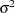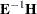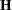Displayed Output

PROC ANOVA first displays a table that includes the following:

• the name of each variable in the CLASS statement

• the number of different values or Levels of the CLASS variables

• the Values of the CLASS variables

• the Number of observations in the data set and the number of observations excluded from the analysis because of missing values, if any

PROC ANOVA then displays an analysis-of-variance table for each dependent variable in the MODEL statement. This table breaks down the Total Sum of Squares for the dependent variable into the portion attributed to the Model and the portion attributed to Error. It also breaks down the Mean Square term, which is the Sum of Squares divided by the degrees of freedom (DF). The analysis-of-variance table also lists the following:

• the Mean Square for Error (MSE), which is an estimate of, the variance of the true errors

• the F Value, which is the ratio produced by dividing the Mean Square for the Model by the Mean Square for Error. It tests how well the model as a whole (adjusted for the mean) accounts for the dependent variable’s behavior. Thistest is a test of the null hypothesis that all parameters except the intercept are zero.

• the significance probability associated with thestatistic, labeled "Pr > F"

• R-Square,, which measures how much variation in the dependent variable can be accounted for by the model. Thestatistic, which can range from 0 to 1, is the ratio of the sum of squares for the model divided by the sum of squares for the corrected total. In general, the larger thevalue, the better the model fits the data.

• C.V., the coefficient of variation, which is often used to describe the amount of variation in the population. The C.V. is 100 times the standard deviation of the dependent variable divided by the Mean. The coefficient of variation is often a preferred measure because it is unitless.

• Root MSE, which estimates the standard deviation of the dependent variable. Root MSE is computed as the square root of Mean Square for Error, the mean square of the error term.

• the Mean of the dependent variable

For each effect (or source of variation) in the model, PROC ANOVA then displays the following:

• DF, degrees of freedom

• Anova SS, the sum of squares, and the associated Mean Square

• the F Value for testing the hypothesis that the group means for that effect are equal

• Pr > F, the significance probability value associated with the F Value

When you specify a TEST statement, PROC ANOVA displays the results of the requested tests. When you specify a MANOVA statement and the model includes more than one dependent variable, PROC ANOVA produces these additional statistics:

• the characteristic roots and vectors offor eachmatrix

• the Hotelling-Lawley trace

• Pillai’s trace

• Wilks’ lambda

• Roy’s greatest root

See Example 41.6 in Chapter 41, The GLM Procedure, for an example of the MANOVA results. These MANOVA tests are discussed in Chapter 4, Introduction to Regression Procedures.